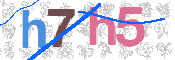Open
X

# 2-Point Measurement

Ohm’s law is used in order to determine a resistance: R=V/I. A known current is sourced and flows through the unknown resistance. We measure the voltage that develops across the resistance by dividing the measured voltage by the sourced current. A problem that occurs when using a 2-wire setup is that the voltage is measured not only across the resistance in question, but also includes the resistance of the leads and contacts . When using an ohmmeter to measure resistances above a few ohms, this added resistance is usually not a problem. However, when measuring low resistances or when contact resistance may be high, obtaining accurate results with a two wire measurement could be a problem.

# 4-Point Measurement

A solution to the problem of 2-point measurements in which the lead and contact resistance is measured along with that of the device under test (DUT) is the 4-wire or “Kelvin” measurement. Because a second set of probes is used for sensing and since negligible current flows in these probes, only the voltage drop across the device under test is measured. As a result, resistance measurement or I-V curve generation is more accurate.